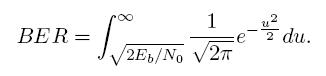# unable to solve this problem - (access points & handoff)

Status
Not open for further replies.

#### yeahboy

##### Newbie level 6
Consider a network consisting of two access points: AP1 and AP2, located at positions (-100,0) and (100, 0) on the plane, respectively, where the distance unit is meter. A mobile unit is communicating through this network, which offers a data rate of 1 Mbps, using BPSK modulation and operating at a frequency spectrum centered around 2.4 GHz. Each access point transmits at the level of 100mW and the noise density in the area is 10?16 W/Hz. Assume that all packets have
the same size: 100 bytes.
(a) Draw a two-dimensional graph that (approximately) indicates the region in which the frame error rate of communication with access point is at least 10%.
(b) Suppose the mobile decides to switch from one access point to another whenever the signal from the newer access point is at least 25% stronger than from the first one. Draw a two dimensional graph that indicates the points at which a mobile will switch from AP1 to AP2.
(c) Repeat part (b) for the case when the mobile decides to switch whenever the signal from the newer access point is at least 25% stronger than from the first one and the frame error rate for communication with the new access point is at most 10%. An approximate curve will suffice.

You may adopt a free-space loss model for idealized isotropic antenna (ignoring gains) and may use the following formula for the Bit-Error-Rate (BER) of BPSK modulation(To calculate the above numerically, you can use the complement of the error function used in statistical analysis { e.g., the ERFC function in Excel.)[/img]

Re: unable to solve this problem - (access points & hand

Here is my solution steps.

1. Mark the points on the plane. It has 4 quadrants. (AP1 on y-axis and AP-2 on x-axis).

2. The problem is to find regions on that space where BER is more than 10%.

3. Choose any point on the plane. Find its distance to AP1 and AP2.

BER when listening to AP1:

Calculate SP: Use free space loss model (lambda/4*pi*d)^2 (i guess)
to calculate signal strength of AP1 and AP2. AP2 signal is interference.
Calculate BER.
Do the samething for AP2. Choose the minimum. If it is more than 10%, the poiny is in the outage region.

Points: 2